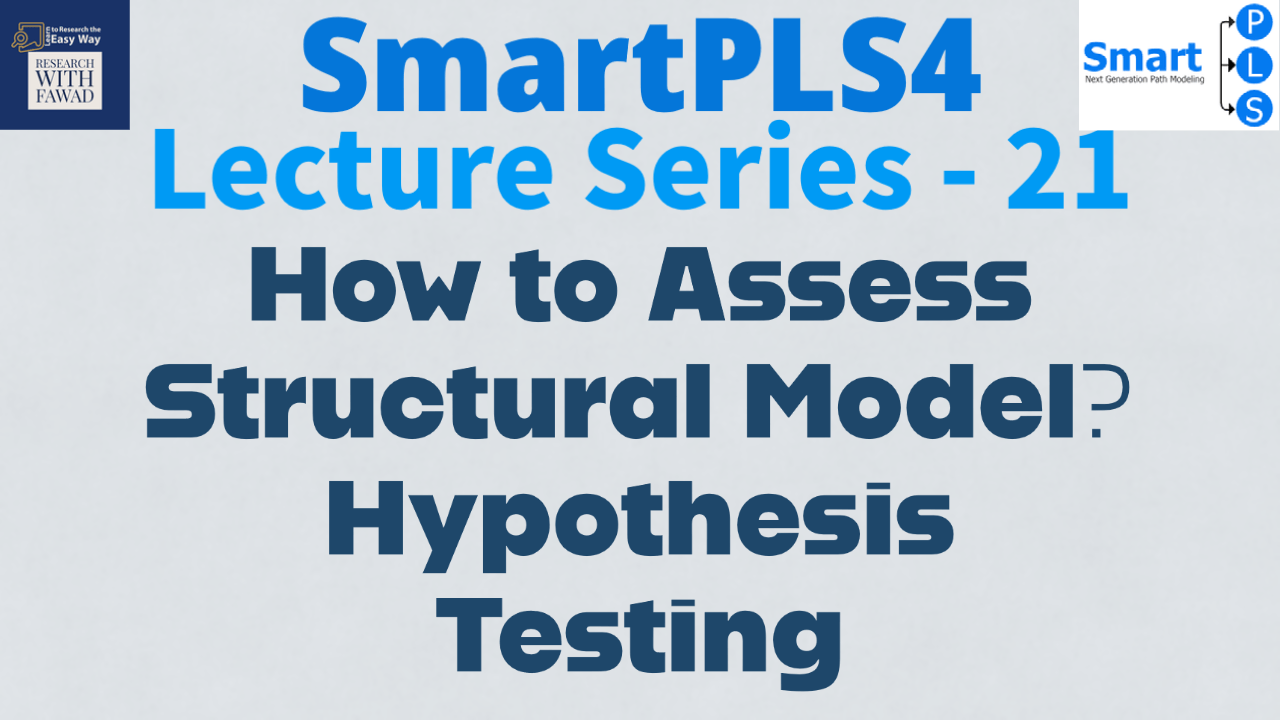# A Simple Structural Model in SmartPLS4

## What is it All About?

The focus of the session is to

• Understand the basic concept of statistics
• Design a simple Structural Model in SmartPLS4### Learn to Analyze a Simple Structural Model in SmartPLS4

The tutorial is a step by step guide on how to design a simple structural model in SmartPLS4.

### What is Beta Coefficient?

The beta coefficient is the degree of change in the outcome variable for every 1-unit of change in the predictor variable.The beta value shows that weight of impact, the standardized beta is in the range of +/-1. The close to 1, the higher is the impact of IV on DV. Normally, a beta value over 0.10 means significant relationship between the variables.

### What is Standard Error?

A measure of the statistical accuracy of an estimate, equal to the standard deviation of the theoretical distribution of a large population of such estimates. In simple terms, It refers to how far is the sample mean from the population mean. The lower it is, the better.

### What is T-Statistics?

It describes how far your observed data is from the null hypothesis of no relationship between variables. A values greater than 1.96 (two-tailed) shows significant relationship.

### What is P Value?

P value is the probability value. The statistics helps establish if the relationship between two variables is significant or not. In social sciences research a p value < 0.05, is referred to as significant. Hence, the hypothesis is supported (the null hypothesis is rejected)